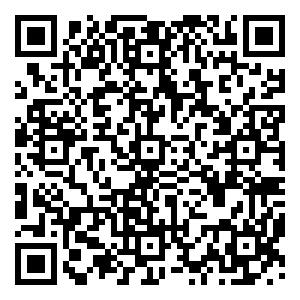## 留言板引用本文: 李华建, 肖作江, 刘颖, 赵媛媛, 王睿智, 贺肖影. 高精度角膜曲率半径测量系统[J]. 中国光学, 2020, 13(3): 501-509.LI Hua-jian, XIAO Zuo-jiang, LIU Ying, ZHAO Yuan-yuan, WANG Rui-zhi, HE Xiao-ying. High precision corneal curvature radius measurement system[J]. Chinese Optics, 2020, 13(3): 501-509. doi: 10.3788/CO.2019-0174
 Citation: LI Hua-jian, XIAO Zuo-jiang, LIU Ying, ZHAO Yuan-yuan, WANG Rui-zhi, HE Xiao-ying. High precision corneal curvature radius measurement system[J]. Chinese Optics, 2020, 13(3): 501-509.## 高精度角膜曲率半径测量系统

##### doi: 10.3788/CO.2019-0174

###### 通讯作者: 肖作江（通讯作者）（1973—），吉林长春人，2003年获得长春理工大学机械设计理论硕士学位，2009年获得长春理工大学光学工程博士学位，现为长春理工大学副研究员、硕士生导师，主要研究方向为精密测量、光电检测。E-mail：xiao2706@163.com
• 中图分类号: TP394.1；TH691.9

## High precision corneal curvature radius measurement system

Funds: Supported by Jilin Province Key Research and Development Project (No. 20180201025GX)
More Information
###### Corresponding author:xiao2706@163.com
• 摘要: 为了实现成像角膜曲率计沿光轴方向的精确对准，提高角膜曲率的测量精度，设计了一种高精度成像角膜曲率测量系统。对该系统的成像光源、成像光学系统、干涉测量系统等进行研究。通过LED阵列均匀照射靶环形成光源；成像物镜采用双远心镜头，使景深变大，有利于对准测量，同时保证成像物镜的放大率不会受景深的影响；引用低相干干涉测量技术，利用低相干干涉信号定位角膜顶点和测量光源的位置，利用光栅尺监测扫描反射镜的位置，实现了角膜顶点到测量光源距离的精确测量。最后，分析了该系统成像物镜放大率的稳定性和角膜曲率测量误差，在理论基础上，做出了实验样机，用所设计的样机对标准角膜模拟眼进行测试，系统精度达到±0.02 mm，基本满足角膜曲率的测量要求。
• 图  1  角膜曲率半径测量原理图

Figure  1.  Measurement principle of corneal curvature radius

图  2  误差分析图

Figure  2.  Error analysis chart

图  3  高精度角膜曲率半径测量系统原理图

Figure  3.  Schematic diagram of measurement system for high precision corneal curvature radius

图  4  靶环装置

Figure  4.  Target ring device

图  5  靶环装置成像原理图

Figure  5.  Schematic diagram of imaging principle of target ring device

图  6  靶环装置组成图

Figure  6.  Composition diagram of target ring device

图  7  靶环装置光源仿真图

Figure  7.  Simulation diagram of light source for target ring device

图  8  模拟面的辐照度

Figure  8.  Irradiances of the simulated surface

图  9  双远心镜头成像原理图

Figure  9.  Imaging principle of double telecentric lens

图  10  干涉测量系统

Figure  10.  Interferometry measurement system

图  11  干涉图

Figure  11.  Interferogram

图  12  $b$为100 μm时角膜曲率半径的误差

Figure  12.  The error of corneal curvature radius when $b$is 100 μm

图  13  $b$为−100 μm时角膜曲率半径的误差

Figure  13.  The error of corneal curvature radius when $b$is −100 μm

图  14  实验样机

Figure  14.  Experimental prototype

图  15  图像处理结果

Figure  15.  Image processing results

表  1  景深对应的$b$$\beta$Table  1.   The $b$and $\beta$values corresponding to different depthes of field

 景深/mm $b$/mm $\beta$/mm −3 67 −0.464 −2 68 −0.459 −1 69 −0.456 0 70 −0.450 1 71 −0.446 2 72 −0.441 3 73 −0.437

表  2  双远心镜头参数

Table  2.   Double telecentric lens parameters

 参数 数值 物距/mm 100 前景深/mm 4.68 后景深/mm 5.47 放大率 –0.45

表  3  不同物距的放大率

Table  3.   Magnifications corresponding to different object distances

 物距/mm 放大率 97 −0.450 19 98 −0.450 15 99 −0.450 12 100 −0.450 00 101 −0.449 92 102 −0.449 86 103 −0.449 82 104 −0.449 78

表  4  实验结果

Table  4.   Experimental results

 标准值 测量值/mm 误差/mm 标准值 测量值/mm 误差/mm 6.656 ${r_{\max }}$ 6.675 ﹢0.019 7.988 ${r_{\max}}$ 7.997 0.009 ${r_{\min }}$ 6.641 −0.015 ${r_{\min}}$ 7.975 −0.013 6.656 ${r_{\max}}$ 6.673 +0.017 9.458 ${r_{\max}}$ 9.466 +0.008 ${r_{\min }}$ 6.637 −0.019 ${r_{\min }}$ 9.443 −0.015 6.656 ${r_{\max}}$ 6.669 +0.013 9.458 ${r_{\max}}$ 9.473 +0.015 ${r_{\min }}$ 6.647 +0.011 ${r_{\min }}$ 9.455 −0.003 7.988 ${r_{\max}}$ 7.999 +0.011 9.458 ${r_{\max}}$ 9.477 +0.019 ${r_{\min }}$ 7.974 −0.014 ${r_{\min }}$ 9.459 +0.001 7.988 ${r_{\max}}$ 7.998 +0.010 ${r_{\min }}$ 7.980 −0.008
•点击查看大图
##### 计量
• 文章访问数:  1086
• HTML全文浏览量:  809
• PDF下载量:  21
• 被引次数: 0
##### 出版历程
• 收稿日期:  2019-08-27
• 修回日期:  2019-11-08
• 刊出日期:  2020-06-01

### 目录/下载:  全尺寸图片 幻灯片
• 分享
• 用微信扫码二维码

分享至好友和朋友圈